NONLINEAR PHENOMENA IN COMPLEX SYSTEMS
An Interdisciplinary Journal

2004, Vol.7, No.2, pp.106-128

Geometry of 3-Spaces with Spinor Structure.
V.M. Red'kov

A special approach to examine spinor structure of 3-space is proposed. It is based on the use of the concept of a spatial spinor defined through taking the square root of a real-valued 3-vector. Two sorts of spatial spinor according to P-orientation of an initial 3-space are introduced: properly vector or pseudo vector one. These spinors,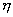and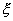, turned out to be different functions of Cartesian coordinates. To have a spinor space model, you ought to use a doubling vector space { (x1,x2,x3 )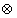(x1,x2,x3)'}. The main idea is to develop some mathematical technique to work with such extended models. Spinor fieldsand, given as functions of Cartesian coordinates xi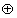xi', do not obey Cauchy-Riemann analyticity condition with respect to complex variable (x1 + i x2)(x1 + i x2)'. Spinor functions are in one-to-one correspondence with coordinates xixi' everywhere excluding the whole axis (0,0,x3)(0,0,x3)' where they have an exponential discontinuity. It is proposed to consider properties of spinor fields(xixi') and(xixi') in terms of continuity with respect to geometrical directions in the vicinity of every point. The mapping of spinor fieldintoand inverse have been constructed. Two sorts of spatial spinors are examined with the use of curvilinear coordinates (y1,y2,y3): cylindrical parabolic, spherical and parabolic ones. Transition from vector to spinor models is achieved by doubling initial parameterizing domain G (y1,y2,y3)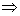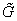(y1,y2,y3) with new identification rules on the boundaries.Different spinor space models are built on explicitly different spinor fields(y) and(y). Explicit form of the mapping spinor field(y) of pseudo vector model into spinor(y) of properly vector one is given, it contains explicitly complex conjugation.
Key words: space, geometry, spinor, continuity, P-orientation, spherical and parabolic coordinates

Full text:  Acrobat PDF  (243KB)   PostScript (490KB)   PostScript.gz (205KB)

Copyright © Nonlinear Phenomena in Complex Systems. Last updated: June 29, 2004